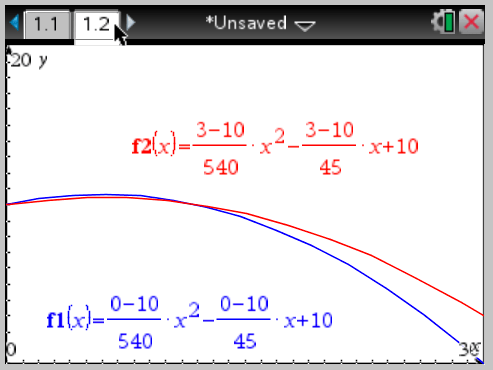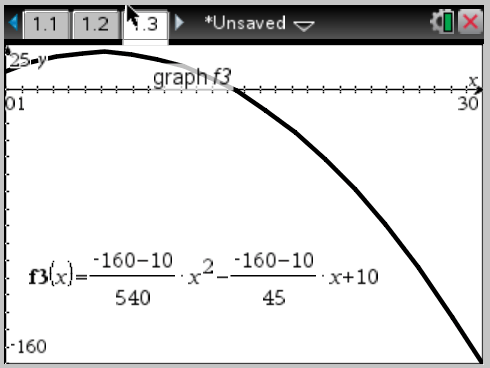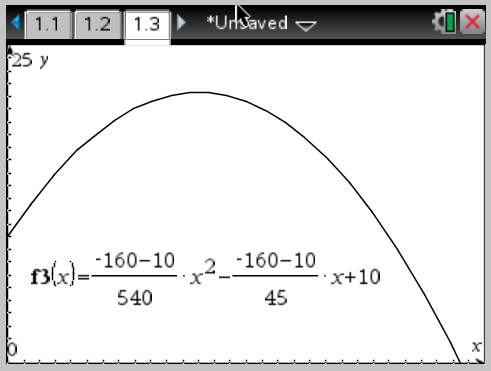Posted on

# A “Problematic” ArcherStudents often bring in questions that are taken or adapted from sources outside of the usual textbooks. This is especially true with regards to revision material when leading up to topic test or other major assessment. One question of this type that really caught my attention was from a "Smart Study" guide given to students preparing for a SAC (i.e. major test) on Functions and Graphs. The diagram is shown here:(note this diagram remains the intellectual property of the copyright holder)

Now as we can plainly see from the diagram, the wall is 10 metres high. The point$P$  is on the wall below the level of point$Q$  and point$S$ . Point$Q$  is also below the top of the wall and point$S$  is almost in line with the top of the wall. The points$Q$  and$S$  are also vertically in line with one another and point$S$  appears to represent the turning point of the parabolic path of the arrow.

The text of the question, however, states that the point$P$  is "10 m above the ground" (i.e. at the top of the wall?). The question text also states that the "edge of the cauldron at$Q$  was 6 m from the wall" and that the cauldron "was 20 m high". Okay, so either the diagram is wrong or the text is wrong (or both)!!

The students are asked to use the information provided, either by the diagram or the text, to determine coordinates for the points$P$ ,$Q$  and$R$ . Clearly this cannot be done accurately from the diagram, so one must assume that the text is the correct information. This leads to the solutions of$P = (0,10)$ ,$Q = (6,20)$  and$R = (30,0)$ . Not a problem (so far).

Next the students are asked to determine the quadratic of the form$y=ax^{2} + bx + c \textrm{, } 0 \leq x \leq 30$  that describes the arrow’s path using the coordinates that they have just found. Whilst it is possible to determine a quadratic using these three points, students cannot find it exactly unless they make some assumption about the release height of the arrow since point$R$  is where the archer is standing. So unless the archer is releasing the arrow from point$R$ , this point is not on the curve. So we should assume that the release point is$(30, h)$ . Equally, the point$Q$  is clearly not on the arrow’s flight path, but it does represent the axis of symmetry. Hence we can show that:

1.$c = 10$  from point$P$
2.$b = -12a$  from point$Q$
3.$h = 540a + 10$  or that$a = (h - 10) \div 540$  from point$R$

It is then possible to determine a range of values for$h$  by using the fact that the point$S$  must have a height in excess of 20 m otherwise the arrow would not fly over the cauldron. Thus we know that the$y$ -coordinate must exceed 20 when$x = 6$ , so we can show that$a$  and$b$  depend on this value of$h$ , but what range of values would be acceptable? Well, common sense tells us that$0 < h < 3$  is a generous range for the release height of the arrow. If we plot graphs at the limits of$h$  we obtain:(note this diagram produced using TI-NSpire™ CAS Teacher Software)

Clearly neither of these graphs achieve an apogee in excess of 20 m and so the release height of the arrow must be in excess of 3 metres if the archer is standing 30 metres away from the wall.

If, instead we work from the assumption that the apogee, which occurs at the turning point ($S$ ), is simply in excess of 20 m we can then solve for$h$ . Since point$S$  is also the turning point, we know that the$y$ -coordinate is given by$-\left(b^{2} - 4ac\right) \div 4a$ . Substituting all the values in terms of$h$  we find that$h < -160$  which makes no physical sense, unless the archer is in a very, very deep pit. If we graph the situation where$h = -160$  we get the following graph:(note this diagram produced using TI-NSpire™ CAS Teacher Software)

Of course, we could have the archer standing closer to the cauldron to ensure that point$R$  was no further away from the wall than the positive$x$ -intercept. This intercept is given by$-\frac{b}{2a} - \left[\sqrt{b^{2} - 4ac} \div 2a\right]$  (remember$a$  is negative) which works out to be$6 - \sqrt{36a^{2}-10a} \div a \approx 14.23$ . Thus if the archer is releases the arrow from a point 0 metres above the ground, they would need to be standing at 14.23m from the wall. To release it from a point higher than 0 metres above the ground, they would need to stand closer to the cauldron (as shown below).(note this diagram produced using TI-NSpire™ CAS Teacher Software)

In all the above calculations, we have assumed that the point$P$  is definitely 10 metres above the ground. If, however, the diagram was correct and the arrow lodges into the wall at a point less than 10 metres above the ground, this would naturally change the results.

The last part of the question asks the students to find the angle$\theta$  "correct to the nearest degree", where$\theta$  is the angle of intersection relative to the horizontal. One assumes that the question author expects the students to approximate the angle of intersection by making a right-angled triangle using points$P$  and$S$ . Of course, a better answer is found using calculus, recognising that$\theta$  is given by the$\arctan$  of the instantaneous slope of the quadratic at point$P$ . Now,$\frac{dy}{dx} = 2ax + b$  and so at point$P$  this is simply the value of$b$ . Hence$\theta = \arctan (b)$ . So once we ascertain appropriate values for$a$ ,$b$  and$c$  we can easily find$\theta$ .

## So what is the problem?

For most students, the mechanics of this question can be completed by ignoring the real-world implications of their solutions. Unfortunately, if any student stops to think about the implications of their solutions, they will reach a point of cognitive dissonance. The mathematics makes sense, but the results don’t match either the diagram or the question text, and thus we come to the real problem. Supposedly, this question is meant to challenge students to solve real-world (i.e. physically possible) problems. Equally, this question is supposed to help students prepare for exams by giving them something that has a unique, but challenging solution. Unfortunately, this question only serves to make students feel that they do not know the material because they either cannot get an answer that fits the supplied data, or they cannot get an answer that makes physical sense.

Without ending up in a rant about how important proof-reading is or equally a rant about how qualified mathematicians should be writing such questions, I will just simply state that teachers should always be careful to set questions for students that they have already PERSONALLY determined the answers for.

First published March 2013 | Last updated: 23 January 2018